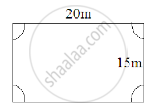Share

# A Rectangular Piece is 20 M Long and 15 M Wide. from Its Four Corners, Quadrants of Radii 3.5 M Have Been Cut. Find the Area of the Remaining Part. - Mathematics

Course
ConceptIntroduction of Mensuration

#### Question

A rectangular piece is 20 m long and 15 m wide. From its four corners, quadrants of radii 3.5 m have been cut. Find the area of the remaining part.

#### Solution

It is given that the length of the rectangular piece is 20 m and its width is 15 m .
And, from each corner a quadrant each of radius 3 . 5 m has been cut out .
A rough figure for this is given below:∴ Area of the remaining part = Area of the rectangular piece - (4 x Area of a quadrant of radius 3 . 5m)
Now, area of the rectangular piece = $20 \times 15 = 300 m^2$
And, area of a quadrant with radius $3 . 5 m =\frac{1}{4} \pi r^2 = \frac{1}{4} \times \frac{22}{7} \times (3 . 5 )^2$
$= \frac{1}{4} \times \frac{22}{7} \times 3 . 5 \times 3 . 5$
$= 9 . 625 m^2$
∴ Area of the remaining part = $300 - (4 \times 9 . 625) = 261 . 5 m^2$

Is there an error in this question or solution?

#### APPEARS IN

RD Sharma Solution for Mathematics for Class 8 by R D Sharma (2019-2020 Session) (2017 to Current)
Chapter 20: Mensuration - I (Area of a Trapezium and a Polygon)
Ex. 20.1 | Q: 4 | Page no. 13
Solution A Rectangular Piece is 20 M Long and 15 M Wide. from Its Four Corners, Quadrants of Radii 3.5 M Have Been Cut. Find the Area of the Remaining Part. Concept: Introduction of Mensuration.
S